Application

# IMU Units in Drones (MEMS triaxial accelerometer, triaxial gyroscope, triaxial magnetometer)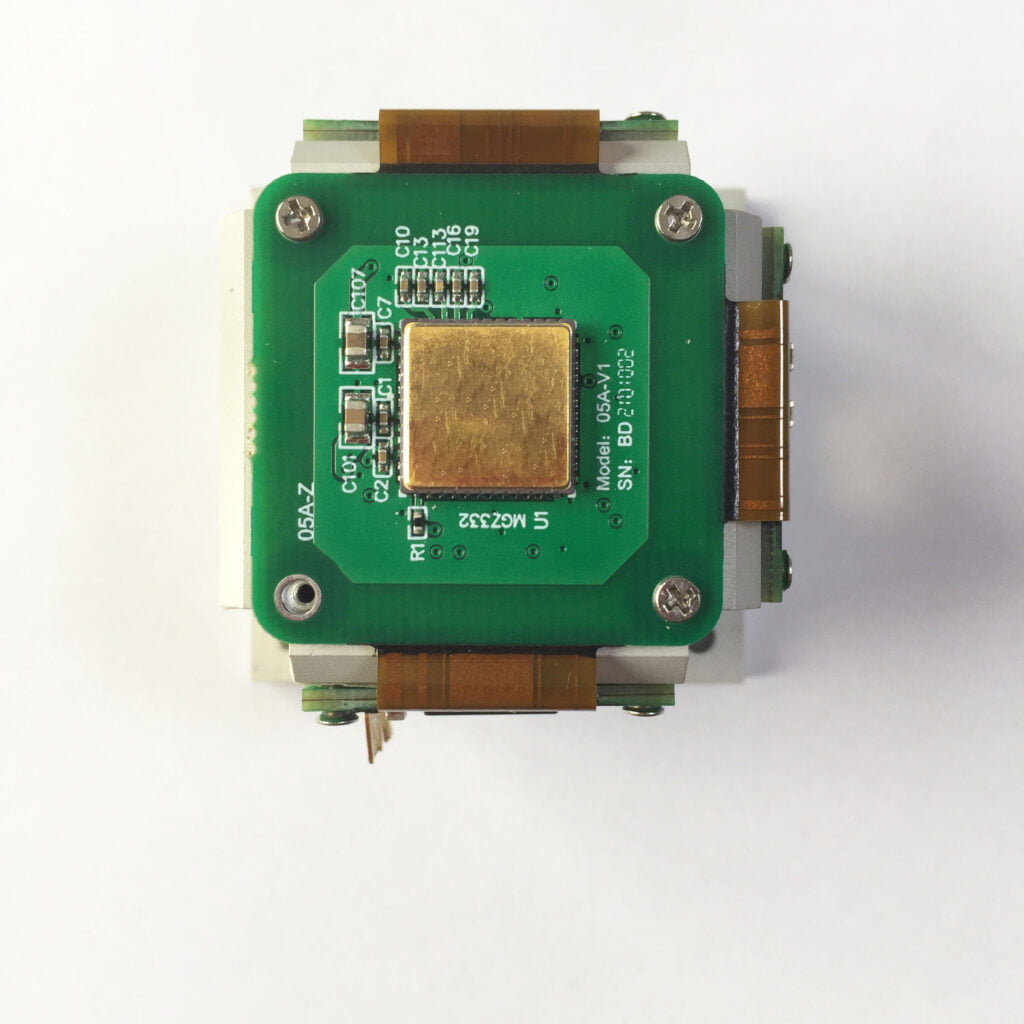Triaxial accelerometer

(1) Measure the specific force

A triaxial accelerometer is an inertial sensor that can measure the specific force of an object, that is, the overall acceleration after removing gravity or the non-gravitational force acting on a unit mass. When the accelerometer remains stationary, the accelerometer is able to sense the acceleration due to gravity, and the overall acceleration is zero. In free fall motion, the overall acceleration is the acceleration of gravity, but the accelerometer is in a weightless state, and the output of the three-axis accelerometer is zero at this time.

(2) Measuring angle

The principle of a triaxial accelerometer can be used to measure angles. Intuitively, as shown, the amount of spring compression is determined by the angle of the accelerometer to the ground. The specific force can be measured by the spring compression length. Therefore, in the absence of external force, the accelerometer can accurately measure the pitch and roll angles without accumulating errors.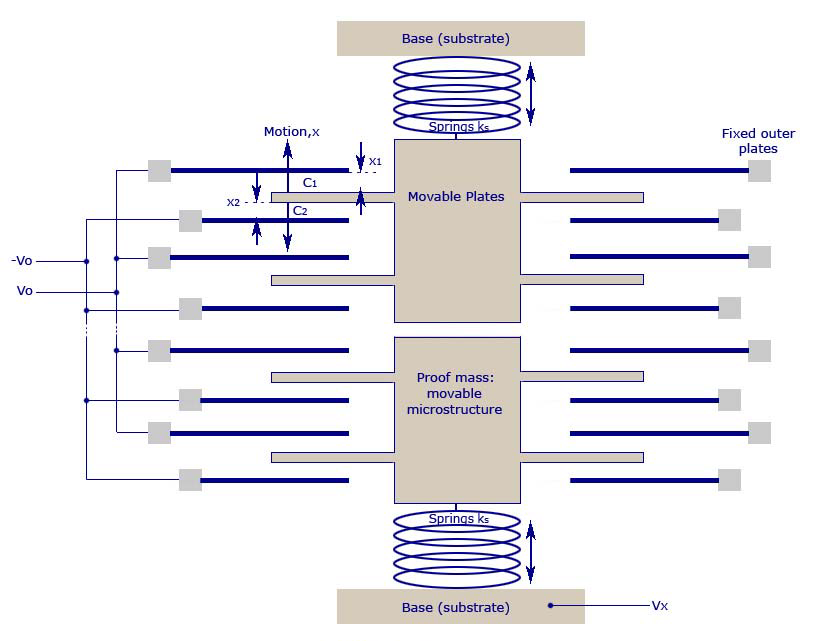MEMS three-axis accelerometers use piezoresistive, piezoelectric and capacitive working principles, and the specific force (pressure or displacement) generated is proportional to the changes in resistance, voltage and capacitance, respectively. These changes can be picked up by corresponding amplification and filtering circuits. The disadvantage of this sensor is that it is greatly affected by vibration.

Between its working principle of measuring angle a three-axis accelerometer cannot measure yaw angle

Can measure pitch and roll angles

Three-axis gyroscope

Function: used for the calculation of the angular velocity in the UAV and the angle after the integration of the angular velocity

Principle: To understand the principle of a three-axis gyroscope, you must first know the Coriolis force

When a particle moves in a straight line relative to the inertial system, its trajectory is a curve relative to the rotating system because of the inertia of the particle itself. Based on the rotating system, we believe that there is a force that drives the trajectory of the particle to form a curve. Coriolis force is a description of this shift, expressed as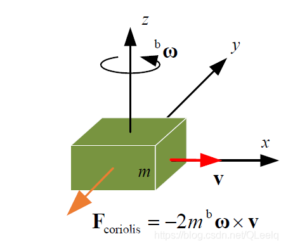That is to say, when the linear motion is placed in a rotating system, the linear trajectory will be offset, but in fact the problem of linear motion is not affected by the force. The establishment of such a virtual force is called the Coriolis force.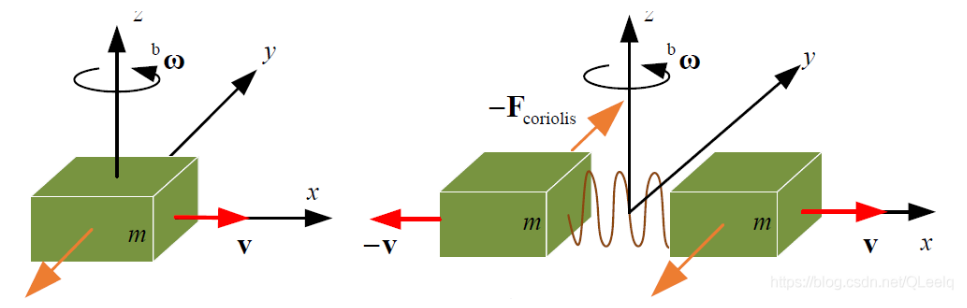Therefore, in the gyroscope, we select two objects, they are in constant motion, and make their motion phase differ by -180 degrees, that is, the two mass blocks move in opposite directions and the same size. The Coriolis force they generate is opposite, thereby compressing the two corresponding capacitive plates to move, resulting in a differential capacitance change. The change in capacitance is proportional to the rotational angular velocity. The rotation angle change can be obtained from the capacitance.

Triaxial magnetometer

The magnetometer can provide the data of the magnetic field that the device is subjected to in each axis of XYZ, and then the relevant data will be imported into the algorithm of the microcontroller to provide the heading angle related to the magnetic north pole, and the geographic orientation can be detected by using this information.

The magnetometer uses three mutually perpendicular magnetoresistive sensors, and the sensor on each axis detects the geomagnetic field strength in that direction.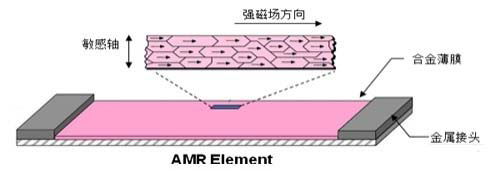The picture above shows an alloy material with a crystal structure. They are very sensitive to the external magnetic field, and the change in the strength of the magnetic field will cause the resistance value of the magnetoresistive sensor to change.

In addition, the three-axis magnetometer can also use the Lorentz force principle. The current flows through the magnetic field to generate force, thereby driving changes such as capacitance.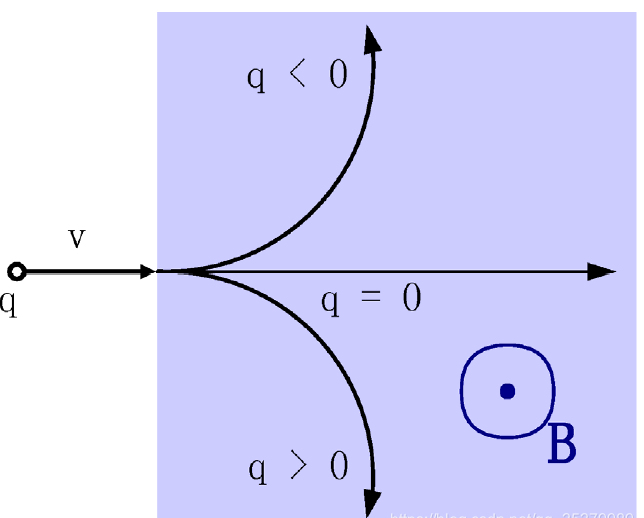imu inertial measurement unit

gyro accelerometer

imu gyroscope

best imu

high accuracy accelerometer